Courses

# Test: Strain Energy & Resilience Level - 1

## 10 Questions MCQ Test Strength of Materials (SOM) | Test: Strain Energy & Resilience Level - 1

Description
This mock test of Test: Strain Energy & Resilience Level - 1 for Mechanical Engineering helps you for every Mechanical Engineering entrance exam. This contains 10 Multiple Choice Questions for Mechanical Engineering Test: Strain Energy & Resilience Level - 1 (mcq) to study with solutions a complete question bank. The solved questions answers in this Test: Strain Energy & Resilience Level - 1 quiz give you a good mix of easy questions and tough questions. Mechanical Engineering students definitely take this Test: Strain Energy & Resilience Level - 1 exercise for a better result in the exam. You can find other Test: Strain Energy & Resilience Level - 1 extra questions, long questions & short questions for Mechanical Engineering on EduRev as well by searching above.
QUESTION: 1

Solution:
QUESTION: 2

Solution:
QUESTION: 3

### Modulus of resilience of a material is the area of the stress-strain curve up to

Solution: Modulus of resilience is the area under the stress strain curve up to the elastic limit.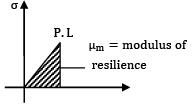QUESTION: 4

Modulus of resilience is defined as

Solution: Modulus of resilience is the proof resilience per unit volume.

QUESTION: 5

For a body of volume V and stresses due to gradually applied load, the strain energy stored is

U =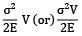QUESTION: 6

The maximum strain energy stored in a circular shaft, under pure torque, (T) is expressed as Umax is

Solution:

U =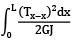U=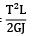QUESTION: 7

Strain energy stored in a solid circular shaft subjected to torsion is proportional to

Solution: Strain energy due to torsion

U =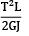∴ U ∝ 1/GJ

QUESTION: 8

The strain energy stored in a body due to suddenly applied load compared to when it is applied gradually is

In sudden applied load, h → 0

If = 1 +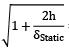= 2

QUESTION: 9

The maximum stress produced in a bar when a load is applied suddenly is 40N/mm2. If the same load is applied gradually, the stress produced is